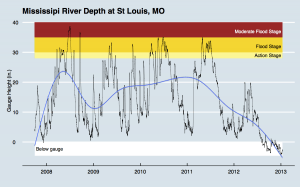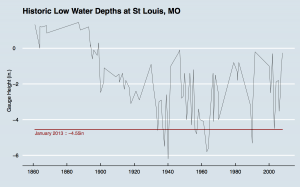Want to share your content on R-bloggers? click here if you have a blog, or here if you don't.

I’ve heard quite a bit about the current problems with the Mississippi River and wanted to see for myself (with data) just how bad it is.

St Louis seems to be quite indicative of the severity of the situation, so I pulled the USGS “stream” records for it and also the historic low water level records for it and put them both into R for some analysis & graphing:click for larger versionclick for larger version

They are both in PDF format as well  

As you can see, there have only been four other (recorded) times when the river was this low and it has just come off of multi-year severely high points with a fairly rapid trend downwards. I’m sure the residents along the Mississippi do not need this data to tell them just how bad things are, but it has helped me understand just how bad the situation is.

For those interested, the R code uses `ggplot2` for time-series charting along with a custom theme and various annotation aids that might be useful to others learning their way around the grammar of graphics in R (so the code is below).

```# # stream.R - graph the recent history of the Mississippi River at St Louis #   library(ggplot2) require(scales) library(ggthemes)   # read in st louis, mo USGS stream data   df.raw = read.csv("~/Desktop/stream.txt")   # need date/time as an R Date for ggplot2 time series plot   df = data.frame(as.POSIXct(df.raw\$datetime,format = "%Y-%m-%d %H:%M"),df.raw\$gauge) df = df[!is.na(df.raw\$gauge),]   # pretty up the column names   colnames(df) = c("datetime","gauge")   # we uses these a few times   maxdate = max(df\$datetime) mindate = min(df\$datetime) mingauge = min(df\$gauge)   # do the plot   st1 = ggplot(df, aes(datetime, gauge)) +   theme_economist() + # pretty theme   # background bands for various water level stages   geom_rect(data=df,aes(xmin=mindate, xmax=maxdate, ymin=28, ymax=30), alpha=1, fill="khaki1") + geom_rect(data=df,aes(xmin=mindate, xmax=maxdate, ymin=30, ymax=35), alpha=1, fill="gold1") + geom_rect(data=df,aes(xmin=mindate, xmax=maxdate, ymin=35, ymax=40), alpha=1, fill="firebrick") +   geom_rect(data=df,aes(xmin=mindate, xmax=maxdate, ymin=mingauge, ymax=0), alpha=1, fill="white") +   # labels for the bands   geom_text(data=data.frame(x=maxdate,y=29), aes(x=x,y=y,label="Action Stage "), size=3, hjust=1) + geom_text(data=data.frame(x=maxdate,y=32), aes(x=x,y=y,label="Flood Stage "), size=3, hjust=1) + geom_text(data=data.frame(x=maxdate,y=37), aes(x=x,y=y,label="Moderate Flood Stage "), size=3, hjust=1, colour="white") +   geom_text(data=data.frame(x=mindate,y=mingauge/2), aes(x=x,y=y,label=" Below gauge"), size=3, hjust=0, colour="black") +   # the raw stream data   geom_line(size=0.15) +   # change the x label to just years   scale_x_datetime(breaks=date_breaks("years"), labels=date_format("%Y")) +   # labels   labs(title="Mississipi River Depth at St Louis, MO", x="", y="Gauge Height (in.)") +   # add a smoothed trend line   geom_smooth() +   # remove the legend   theme(legend.position = "none")   # make a PDF   ggsave("~/Desktop/mississippi.pdf",st1,w=8,h=5,dpi=150)```
```# # low.R - graph the historic low records for the Mississippi River at St Louis #   library(ggplot2) require(scales) library(ggthemes)   # read in historic low records   df.raw = read.csv("~/Desktop/low.csv")   # need date/time as an R Date for ggplot2 time series plot   df = data.frame(as.POSIXct(df.raw\$date,format = "%m/%d/%Y"),df.raw\$gauge) colnames(df) = c("date","gauge")   # pretty up the column names   maxdate = max(df\$date) mindate = min(df\$date)   # do the plot   low1 = ggplot(data=df,aes(date,gauge)) + geom_rect(data=df,aes(xmin=mindate, xmax=maxdate, ymin=-4.55, ymax=-4.55), alpha=1, color="firebrick",fill="firebrick") + geom_text(data=data.frame(x=mindate,y=-4.75), aes(x=x,y=y,label="January 2013 :: -4.55in"), size=3, hjust=0, colour="firebrick") + geom_line(size=0.15) + labs(title="Historic Low Water Depths at St Louis, MO", x="", y="Gauge Height (in.)") + theme_economist()   ggsave("~/Desktop/low.pdf",low1,w=8,h=5,dpi=150)```